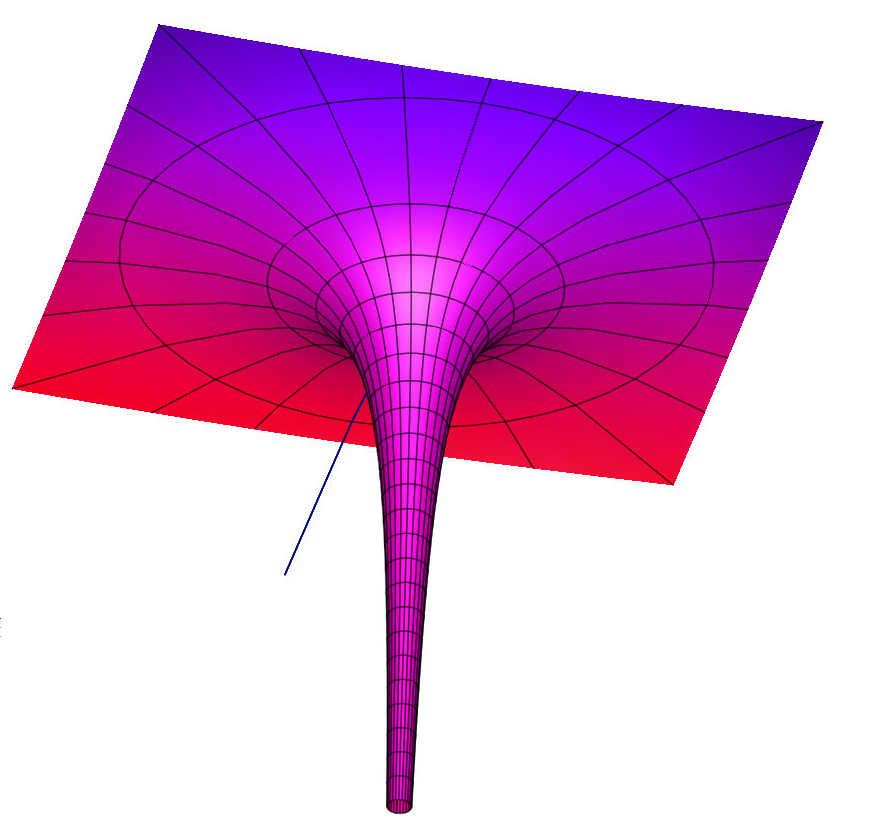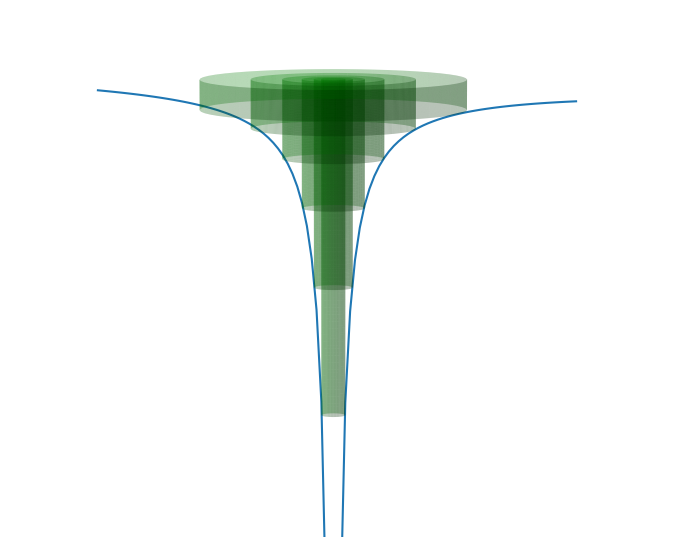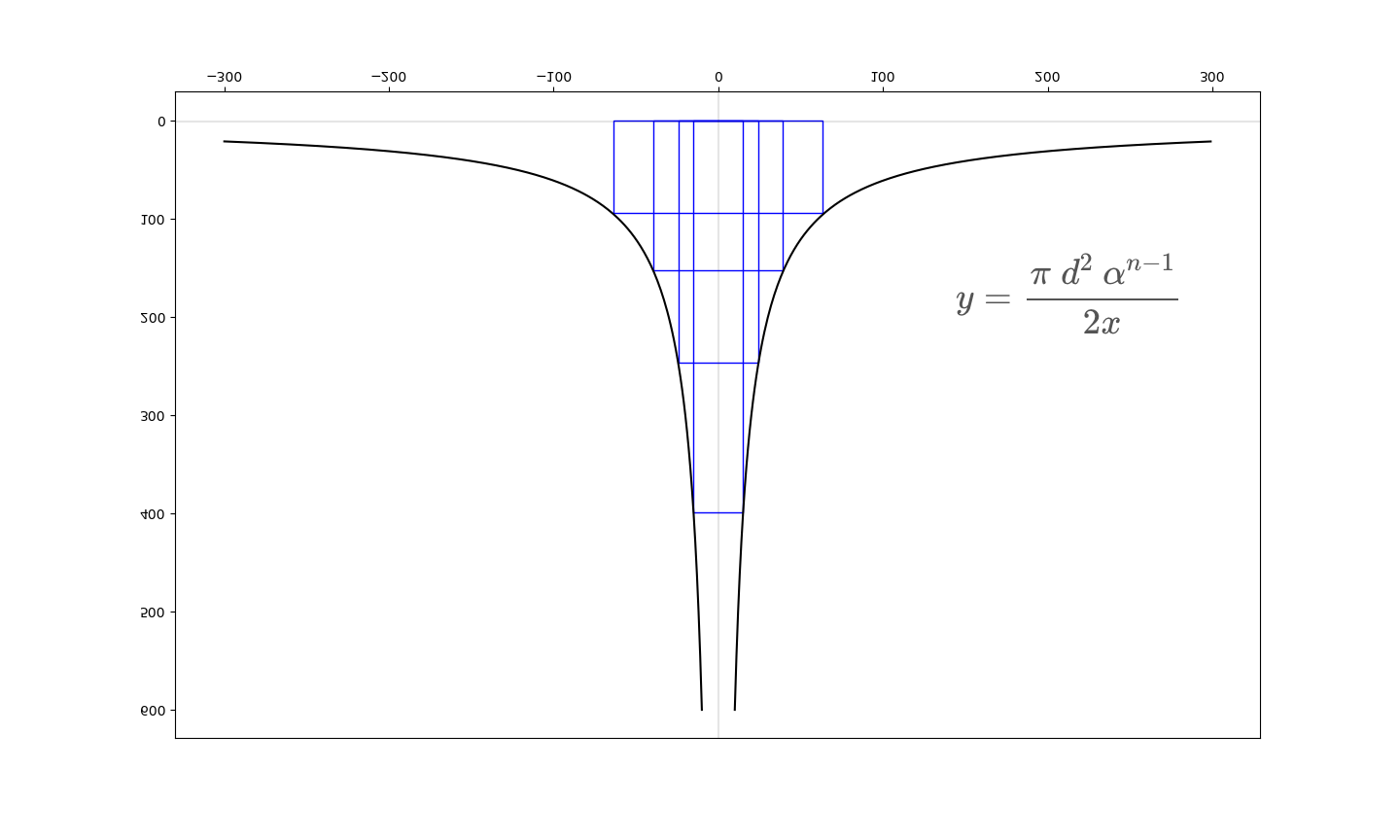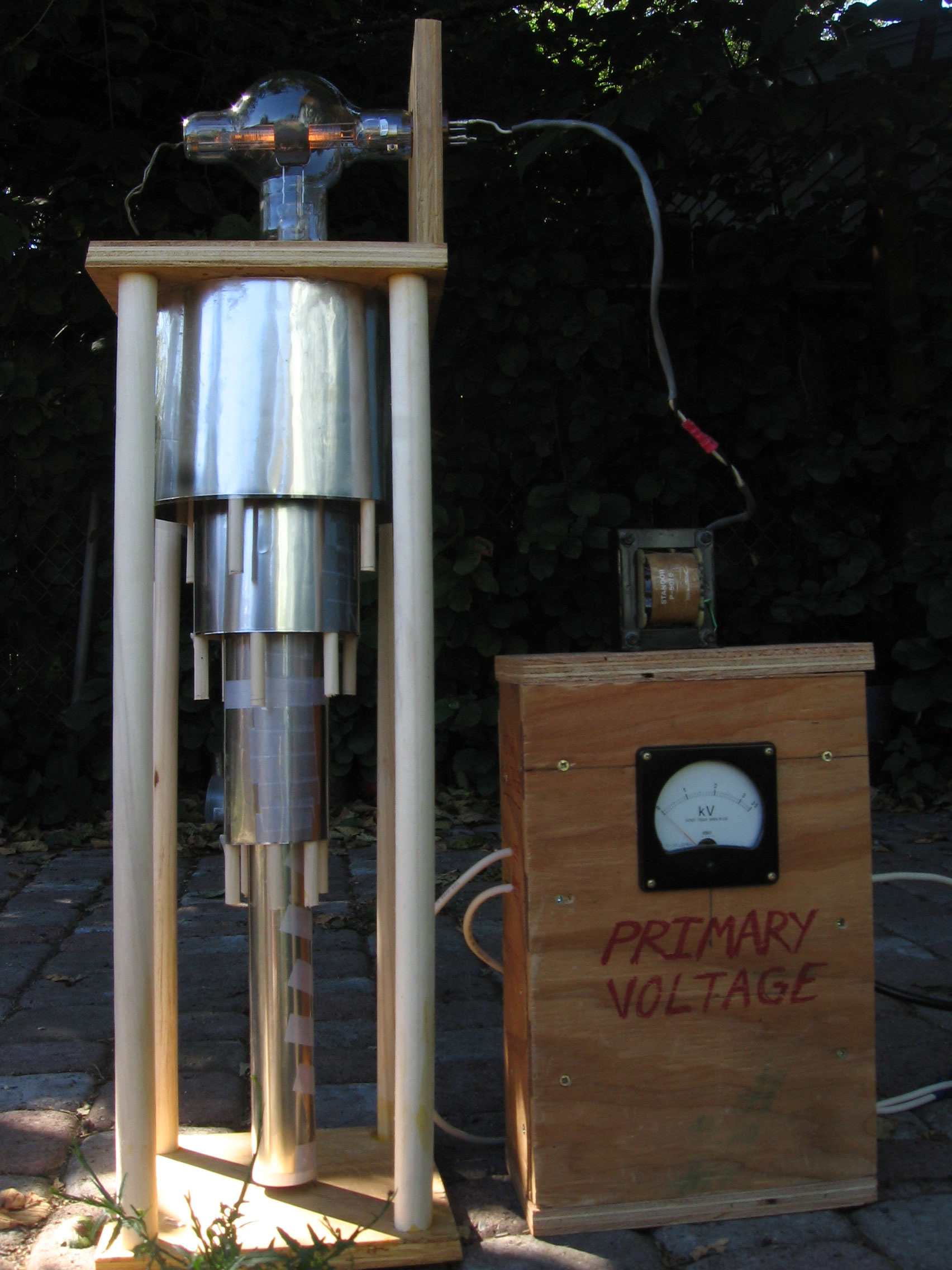# Hyperbolicum AcutumThe Equation for the Hyperbola is: $$y = \frac{ \pi \; d^2 \; \alpha^{n-1}}{2x}$$ Where:
d = Diameter of Innermost Cylinder
alpha = 1.61803... the Divine Proportion
n = Number of Cylinders

The Hyperbola is rotated around the Y-Axis to form the Hyperbolicum Acutum. The Y-Axis runs down the center of the innermost cylinder.

The Hyperbolicum Acutum is the form created by a vortex in water.

In a vortex, the angular velocity of the water is inversely proportional to the distance from the center.

A vortex may look like a spiral, but the medium actually moves in concentric circles. The spiral you see, like the spiral arms of our galaxy, is an optical illusion caused by the stars near the center of the galaxy orbiting the galactic core in less time than the stars further out.

The Vorticity of a fluid flow is a similar idea to that of a Magnetic Field. Both the Vorticity, omega, and the Magnetic Field, B, are defined as the curl of a vector field. When Maxwell wrote his equations, he imagined a fluid flowing and circulating. A fluid he called the AEther. $$\require{physics}$$ $$\vb{\omega} = \curl \vb{u}$$ $$\vb{B} = \curl \vb{A}$$

1. Edwards, Lawrence, “The Vortex of Life: Nature’s Patterns in Space and Time”. Edinburgh: Floris Books, 1993.
2. Maxwell, James Clerk (1865). "A dynamical theory of the electromagnetic field". Philosophical Transactions of the Royal Society of London. 155: 459–512